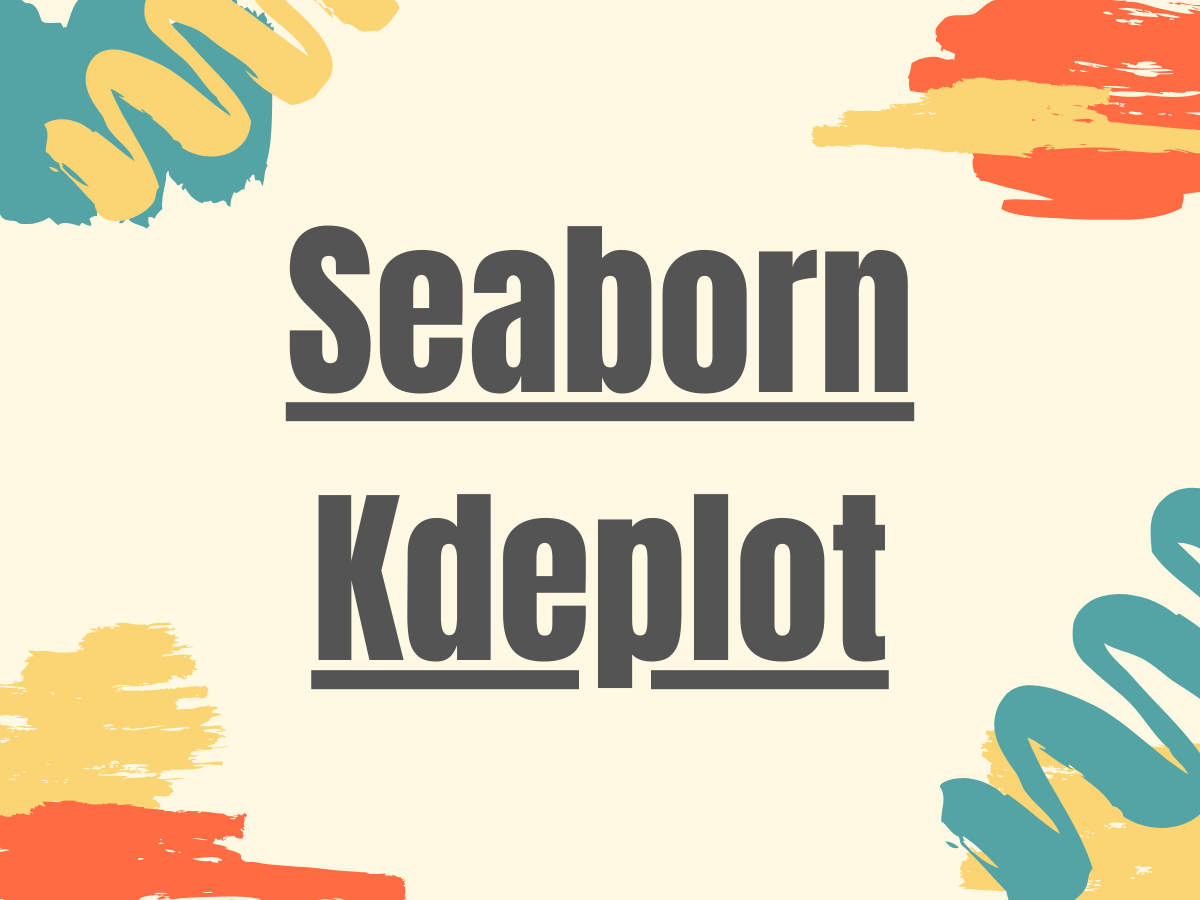# Seaborn Kdeplot - A Comprehensive Guide

Published on August 3, 2022By Safa MulaniWhile we believe that this content benefits our community, we have not yet thoroughly reviewed it. If you have any suggestions for improvements, please let us know by clicking the “report an issue“ button at the bottom of the tutorial.

Hey, folks! In our Seaborn tutorial, we will be focusing on Seaborn Kdeplot.

## What is Kdeplot?

`Kdeplot` is a Kernel Distribution Estimation Plot which depicts the probability density function of the continuous or non-parametric data variables i.e. we can plot for the univariate or multiple variables altogether. Using the Python Seaborn module, we can build the Kdeplot with various functionality added to it.

In order to use the Seaborn module, we need to install and import the module using the below command:

``````pip install seaborn

import seaborn
``````

## Creating a Univariate Seaborn Kdeplot

The seaborn.kdeplot() function is used to plot the data against a single/univariate variable. It represents the probability distribution of the data values as the area under the plotted curve.

Syntax:

``````seaborn.kdeplot(data)
``````

Example 1:

``````import seaborn as sn
import matplotlib.pyplot as plt
import numpy as np
data = np.random.randn(200)
res = sn.kdeplot(data)
plt.show()
``````

In the above example, we have generated some random data values using the numpy.random.randn() function.

Output:

Example 2:

``````import seaborn as sn
import matplotlib.pyplot as plt
import numpy as np
data = np.random.randn(200)
plt.show()
``````

In the above example, we have highlighted the plot using the parameter - ‘`shade`’ to highlight the area under the curve. Further, we can set different colors to the plot using the parameter - ‘`color`’.

Output:

## Creating a Bivariate Seaborn Kdeplot

Seaborn Kdeplots can even be used to plot the data against multiple data variables or bivariate(2) variables to depict the probability distribution of one with respect to the other values.

Syntax:

``````seaborn.kdeplot(x,y)
``````

Thus, the distribution is represented as a contour plot depicting the relationship of the distribution between the two data variables.

Example:

``````import seaborn as sn
import matplotlib.pyplot as plt
import numpy as np
import pandas
plt.show()
``````

Output:

## Plotting Seaborn Kdeplot along the Vertical axis

We can plot the Kdeplots along the y-axis using the below syntax:

Syntax:

``````seaborn.kdeplot(data,vertical=True)
``````

Thus, by setting the ‘`vertical`’ parameter to True, we can plot the distribution against the y-axis.

Example:

``````import seaborn as sn
import matplotlib.pyplot as plt
import numpy as np
import pandas
plt.show()
``````

Output:

## Using color palettes within a Seaborn Kdeplot

Different color palettes can be used along with the Seaborn plots to visualize the data in a better manner using the ‘`cmap`’ parameter.

Different types of color palettes are available at Matplotlib Colormap.

Syntax:

``````seaborn.kdeplot(data,cmap)
``````

Example:

``````import seaborn as sn
import matplotlib.pyplot as plt
import numpy as np
import pandas
plt.show()
``````

Output:

## Plotting two shaded Bivariate Kdeplots

The two shaded Bivariate Kdeplots help in understanding the variation of the data in terms of the probability distribution of the bivariate group of data variables.

Example:

``````import seaborn as sn
import matplotlib.pyplot as plt
import numpy as np
import pandas
sn.set(style='dark',)
plt.show()
``````

Output:

## Addition of a Colorbar to a Seaborn Kdeplot

A `colorbar` maps the pictorial representation of values against the original data values and helps visualize the data in a better manner.

Syntax:

``````seaborn.kdeplot(data,cbar=True)
``````

Example:

``````import seaborn as sn
import matplotlib.pyplot as plt
import numpy as np
import pandas
sn.set(style='dark',)
plt.show()
``````

Output:

## Conclusion

Seaborn module is purely built upon the Matplotlib module and the combination is extensively used to visualize the data in different forms.

I would strongly recommend the readers to go through Python Matplotlib Tutorial for a better understanding about the basics of data visualization.

Click below to sign up and get \$200 of credit to try our products over 60 days!

### Popular Topics

##### Join the Tech Talk##### Hollie's Hub for Good

Working on improving health and education, reducing inequality, and spurring economic growth? We’d like to help.